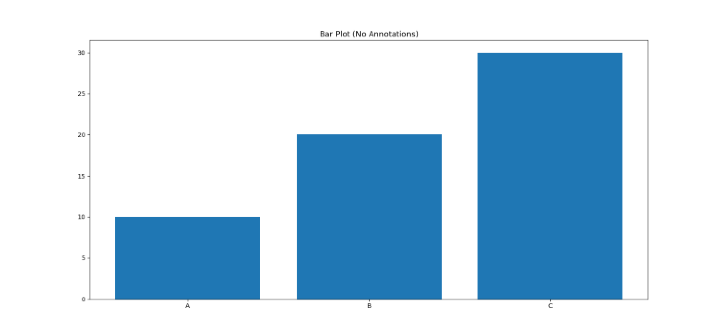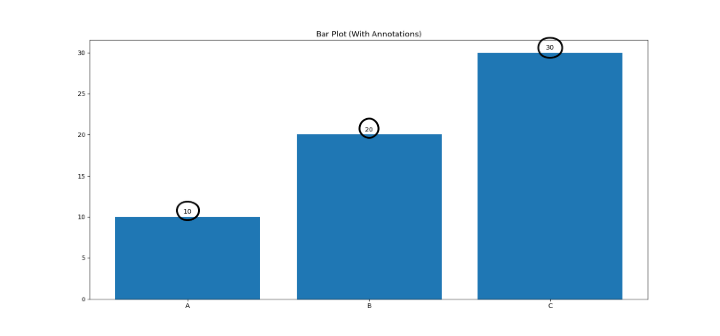# How To Annotate Bars in Bar Plot with Matplotlib in Python?

## Introduction

Bar plots are a common sort of chart used in data visualization. They are a go-to choice for many data scientists since they are easy to produce and comprehend. Bar charts, however, might fall short when we need to visualize additional information.

Annotations are useful in this situation. In bar plots, annotations may be used in order to better comprehend the data.

## Syntax and Usage

Use Matplotlib's annotate() function. The method accepts a number of inputs, such as the text to annotate, where the annotation should be placed, and several formatting choices including font size, color, and style. The annotate() function's fundamental syntax is as follows &minnus;

ax.annotate(text, xy, xytext=None, arrowprops=None, **kwargs)

• text − the text string to be displayed as the annotation

• xy − the (x, y) coordinates of the point to be annotated

• xytext − the (x, y) coordinates of the text position. If not specified, xy will be used.

• arrowprops − a dictionary of arrow properties such as color, width, style, etc.

• **kwargs − extra keyword arguments for styling the annotation text, such as font size, color, and so on.Certain data points can be labeled or more information can be added to a plot using the annotate() method. Moreover, it may be used to produce graphical components like arrows or other markers that indicate particular plot points.

To annotate bars in a bar plot with Matplotlib, we can make use of this algorithm −

• Import the necessary libraries

• Create a figure object using plt.figure().

• Create the bar plot using ax.bar().

• Loop through the bars and add annotations using ax.annotate().

• Pass the height, width and the text to display to the annotate() function

• Render the figure using plt.show()

## Example

import matplotlib.pyplot as plt

# Create a figure object
fig = plt.figure()

# Add a subplot to the figure

# Create the bar plot
bars = ax.bar(['A', 'B', 'C'], [10, 20, 30])

# Loop through the bars and add annotations
for bar in bars:
height = bar.get_height()
ax.annotate(f'{height}', xy=(bar.get_x() + bar.get_width() / 2, height), xytext=(0, 3),
textcoords="offset points", ha='center', va='bottom')

# Show the plot
plt.title('Bar Plot (With Annotations)')
plt.show()

• Begin by making a figure object and attaching a subplot to it. Then, use the plt.bar() method to generate the bar plot, and the resulting bars are saved in a variable called bars. Cycle through the bars and use the plt.annotate() method to add annotations.

• The first option is the text that you wish to annotate, which in this case is the height of the bar. The xy parameter is then used to indicate the position of the annotation, which is a (x, y) coordinate pair.

• The xytext option is used to indicate the text's offset from the xy coordinate. Lastly, use the ha and va options to specify the horizontal and vertical alignment of the text.

• It's worth noting that the plt.annotate() method gives you a number of options for customizing the annotations in your bar graphs. You may design annotations that are exactly matched to your individual needs by experimenting with different values for the xy, xytext, ha, and va variables.

## Conclusion

You may add unique annotations to your bar plots in Matplotlib that assist in explaining the data being presented by using the annotate() function. This article outlines a step-by-step algorithm that will make it simple for you to add this functionality to your own applications. You may make useful and aesthetically pleasing bar plots with comments by simply following the instructions.